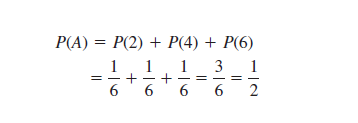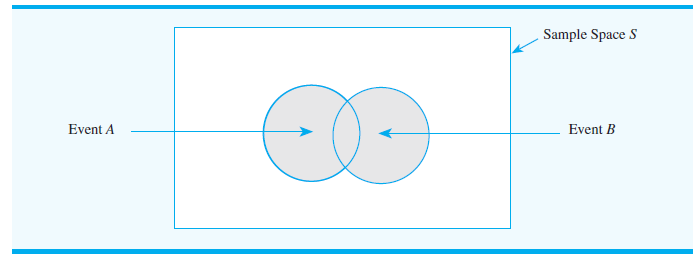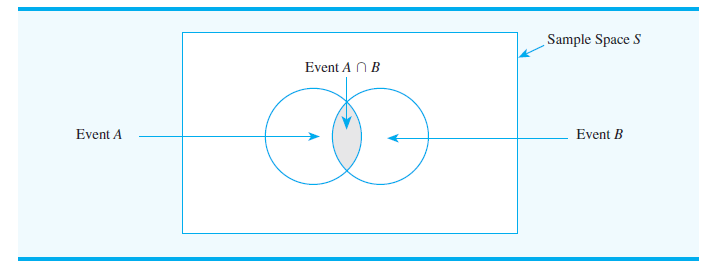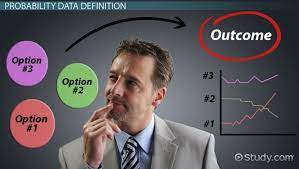I made a commitment to regularly blog about my class sessions on mathematics and noticed that it has become a means for me to revise my learning in class. Going back to the class recordings, my notes and online research have proven very useful. I have a better understanding of what is being taught in class. Anyway, I am sure the title of this post already speaks to what it’s about. I will be sharing my learning from the probability class. In this post, I will discuss on two sections:

1. Events and their probabilities.
2. Combination of events.

## Events and their probability

To recap from my previous post, an event is a collection of sample points. An example is in the experiment of rolling a standard die, the sample space has six sample points and is denoted as D  {1, 2, 3, 4, 5, 6}.

To better understand, in rolling a dice, the probability of rolling a 2 is 1/6, the probability of rolling a 4 is 1/6, and the probability of rolling a 6 is 1/6. The probability of an even number to face upward face of a die is P(D) = P(2)  P(4)  P(6)Any time that we can identify all the sample points of an experiment and can assign the corresponding sample point probabilities, we can use the above method to calculate the probability of an event.

## Union of events

The union of two or more sets is the set that contains all the elements of the two or more sets. Union is denoted by the symbol ∪. For two events A and B, the union of events A and B is the event containing all sample points belonging to A or B or both. The union is denoted A ∪ B. Below is a Venn diagram that shows the union of events A and B. The shaded region contains all the sample points in event A and all the sample points in event B. The fact that the circles overlap indicates that some sample points are contained in both A and B.The general probability addition rule for the union of two events states that P(A∪B)=P(A)+P(B)−P(A∩B), where A ∩ B  is the intersection of the two sets. If the sets are disjoint it can be written as P (A U B) = P(A) + P (B)

## Intersection of events

The intersection of two or more sets is the set of elements that are common to every set. The symbol  ∩  is used to denote the intersection. For two events A and B, the intersection of events A and B is the event containing the sample points belonging to both A and B. The intersection is denoted by A ∩ B. The Venn diagram below shows the intersection of the two events. The area where the two circles overlap is the intersection; it contains the sample points that are in both A and B.When events are independent, we can use the multiplication rule for independent events, which states that P(A∩B)=P(A) P(B)

## Personal thoughts

Thanks to revision by blogging, I am getting the hang of probability. I am at ease when it is time for the data analytics class.## Next Generation Fixed Wireless Access (NGFWA) for Service Providers: A Viable Alternative to Traditional Fiber-Optic Networks

in## Transforming Nigeria’s Local Government System for Rural Economic Growth and Increased GDP

in